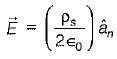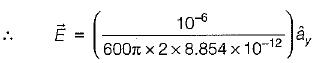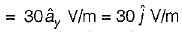Courses

# Electrostatics -2

## 20 Questions MCQ Test Topicwise Question Bank for Electrical Engineering | Electrostatics -2

Description
This mock test of Electrostatics -2 for Electrical Engineering (EE) helps you for every Electrical Engineering (EE) entrance exam. This contains 20 Multiple Choice Questions for Electrical Engineering (EE) Electrostatics -2 (mcq) to study with solutions a complete question bank. The solved questions answers in this Electrostatics -2 quiz give you a good mix of easy questions and tough questions. Electrical Engineering (EE) students definitely take this Electrostatics -2 exercise for a better result in the exam. You can find other Electrostatics -2 extra questions, long questions & short questions for Electrical Engineering (EE) on EduRev as well by searching above.
QUESTION: 1

### Which one of the following pairs is NOT correctly matched?

Solution:

Option (c) is Faraday's law given by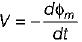QUESTION: 2

Solution:
QUESTION: 3

### What is the value of total electric flux coming out of a closed surface?

Solution:

From Gauss’s law, total electric flux from a closed surface is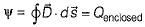.

QUESTION: 4

Which one of the following is the Poisson's equation for a linear and isotropic but in homogeneous medium?

Solution:

For homogeneous medium ∇2 V = -ρ∈ and for non-homogeneous medium ∈ is a variable quantity due to which Poisson’s equation becomes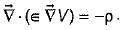QUESTION: 5

Two concentric spherical shells carry equal and opposite uniformly distributed charges over their surfaces as shown in figure below.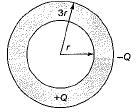Electric field on the surface of inner shell will be

Solution:
QUESTION: 6

Electric field intensity due to line charge of infinite length is

Solution:
QUESTION: 7

Consider the following statements associated with equipotential surface:
1. Potential is same everywhere.
2. No current flows on this surface.
3. Work done in moving charge from one point to another is zero.
4. Potential is different everywhere.
Which of the above statements is/are not correct?

Solution:

Potential is same everywhere on an equipotential surface. Hence, statement 4 is not correct. Statements 1,2 and 3 are correct.

QUESTION: 8

A finite sheet 0 ≤ x ≤ 1, 0 ≤ 1 on the z = 0 plane has a charge density
ρs = xy (x2 + y2 + 25)3/2 nC/m2.
The total charge on the sheet would be

Solution:

The total charge on the sheet is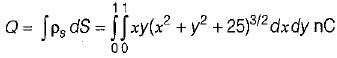Since,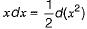, we now integrate w.r.t. x
(or change variables: x2 = u so that xdx = du/2)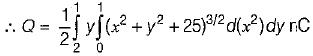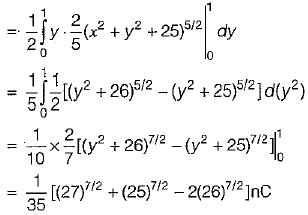QUESTION: 9

Assertion (A): Gauss’s law is an alternative statement of Coulomb’s law.
Reason (R): Proper application of the divergence theorem to Coulomb’s law results in Gauss’s law.

Solution:
QUESTION: 10

If the potential V = 10/r2 sinθcosϕ then the work done in moving a 10 μC charge from point A(1, 30°, 120°) to B(4, 90°, 60°) would be equal to

Solution:

Required work done,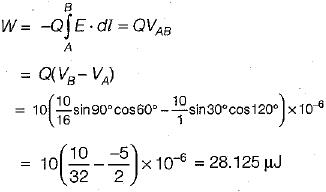QUESTION: 11

A wire of diameter 1 mm and conductivity 5 x 107S/m has 1029 free electrons /mwhen an electric field of 10 mV/m is applied.
The current density and the drift velocity of the electrons will be respectively given by (take charge on an electrons e = -1.6 x 10-19 C)

Solution:

The charge density of free electron is:
ρv = ne = (1029) x (-1.6 x 10-19)
= -1.6 x 1010 C/m2
Given,
E = 10 mV/m,
d = 1 mm,
σ = 5 x 107 S/m,
n = 1029/m3
Now, current density,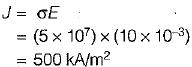Also, drift velocity,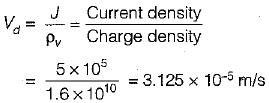QUESTION: 12

The equivalent capacitance between the terminals A and B for the capacitor shown below would be (the area occupied by each dielectric is 30 cm2)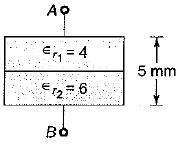Solution:

Given,
d = 5 mm, d/2 = 2.5 mm,
f1 = 4, ∈f2 = 6,
A/2 = 15 cm2
For the given arrangement of dielectrics, D and E are parallel to the dielectric interface. Therefore, we can treat the capacitor as consisting of two capacitors C1 and C2 in parallel (same voltage across C1 and C2).
Thus,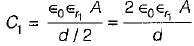and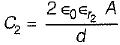The total capacitance/equivalent capacitance between terminals A and B is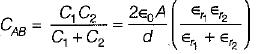QUESTION: 13

Three point charges -1 nC, 4 nC and 3 nC are located at (0, 0, 0) , (0,0,1) and (1,0,0,) respectively. To total energy contained in the system would be

Solution:

Total energy contained in the system is:
W=W+ W+ W3
= 0 + Q2V21 + Q3 (V31 + V32)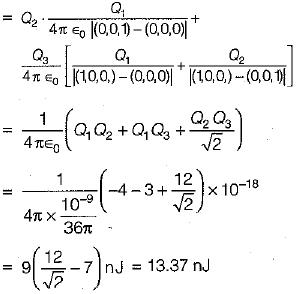QUESTION: 14

The cylindrical coordinates equation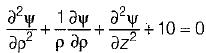is called

Solution:
QUESTION: 15

Image theory is applicable to problems involving

Solution:
QUESTION: 16

Match List-I with List-ll and select the correct answer using the codes given below the lists:
List-I
A. Joule’s law
B. Ohm’s law
C. Solenoidal current
D. Continuity equation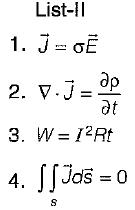Codes:
A B C D
(a) 4 2 3 1
(b) 3 1 2 4
(c) 3 1 4 2
(d) 4 3 1 2

Solution:
QUESTION: 17

Assertion (A): An electric field produces no migration of charge in a dieiectric.
Reason (R): The permittivity of a dielectric is always less than the permittivity of vaccum.

Solution:
• Assertion is a true statement.
• Reason is a false statement because the permittivity of dielectric is always more than the permittivity of vaccum which makes it a good insulator.
• In a dielectric or insulator the electrons are so tightly bound by their parent nuclei in the equilibrium positions that they can not easily be detached by the applications of electric field. Due to this reason an electric field produces no migration of charge in a dielectric.
QUESTION: 18

The potential field at any point in space containing a dielectric material of relative permittivity 3 is given by V = (5x2y + 3yz2 + 6xz) Volts, where x, y, z are in meters. The magnitude of volume charge density at point (1,2, 3) will be given by

Solution:

Given, V = (5x2y + 3yz2 + 6xz) Volts and
r = 3
Using Poisson’s equation,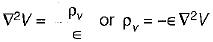Here,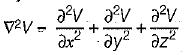= 10y + 0 + 6y = 16y
So,  ρv = -∈ (10y+6y)
∴  ρv|at (1,2,3) = -∈0r (10 x 2 + 6 x 2)
= -∈0 x 3 x 32 = - 96∈0
= 96∈0 (Magnitude only)

QUESTION: 19

If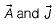are the vector potential and current density vectors associated with a coil, then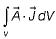has the unit of

Solution:

On comparing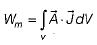with electrical energy stored i.e.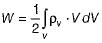we can find that Wm has the unit of energy.

QUESTION: 20

In the infinite plane, y = 6 m, there exists a uniform surface charge density of (1/600π) μC/m2 . The associated electric field strength is

Solution:

Given, y = 6 cm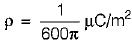The electric field strength due to an infinite charged sheet is given by